### How satellites move in space

• A satellite’s orbit works because of a balance between two forces viz.
• Satellite’s velocity – the speed it is travelling in a straight line
• Force of the Earth’s gravitational pull on the satellite
• An artificial satellite is always falling towards earth, but it has enough tangential velocity to continue fall indefinitely.
• Centripetal force on the satellite balances the gravitational attractive force of the earth.
• This balance does not depend upon the mass and size of the satellite.

### Orbit of a Satellite

• Before designing a satellite, scientists choose an orbit that is appropriate to its function as a satellite as one in an orbit very high up will not be able to see objects on Earth in as much detail as orbits that are lower, and closer to the Earth’s surface.
• Similarly, the speed of the satellite moving in the orbit, the angle over the Earth the satellite takes, the areas which the satellite can observe, and the frequency with which the satellite passes over the same portions of the Earth are all important factors to consider when choosing an orbit.
• Finally, the place from which a satellite is launched is important in determining its orbit.
• A satellite launched from a place with higher latitude would have a higher angle of inclination, suitable to launch satellites into polar orbits
• To put a satellite into equatorial orbit, it would be best to launch it from somewhere close to the equator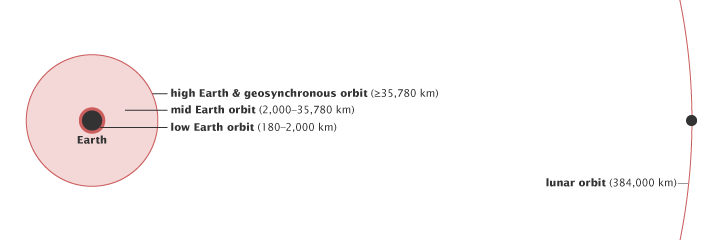### International Space Station

• a space station / a habitable artificial satellite, in low Earth orbit
• the largest artificial body in orbit and can often be seen with the naked eye from Earth
• suited for the testing of spacecraft systems and equipment required for missions to the Moon and Mars
• serves as a space environment research laboratory in which crew members conduct experiments

## Types of Satellite Orbits

• Majorly, there are two major divisions in orbit types; viz. circular orbits and elliptical orbits
• Circular orbits comprises of geostationary, polar, sun-synchronous, and equatorial orbits

#### Circular Orbit

• Though no orbit is perfectly circular, the general name for any orbit that is not highly elliptical is circular
• Circular orbits have an eccentricity of 0
• The orbital path of satellites in these circular orbits is also affected by the satellite’s altitude – its height above the Earth

• Satellites in geostationary orbit are always in a high orbit.
• If the satellite is in a polar, sun-synchronous, or equatorial orbit, its orbital altitude may be medium or it may be low

#### Equatorial Orbit

• Moves along the line of the Earth’s equator
• To get into equatorial orbit, a satellite must be launched from a place on Earth close to the equator
• Equatorial orbits can be useful for satellites observing tropical weather patterns, as they can monitor cloud conditions around the globe.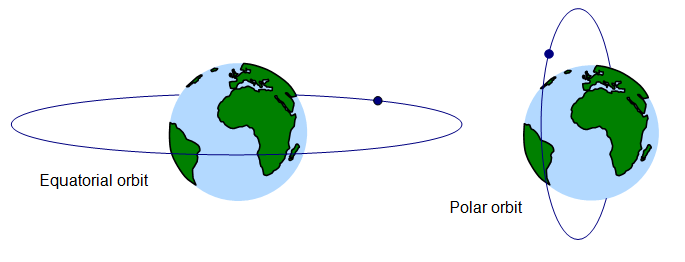#### Geosynchronous orbit

• Has a period of revolution is equal to period of rotation of earth, but its orbit is not equatorial with anorbital period of one sidereal day, matching the Earth’s sidereal rotation period
• synchronized with the Earth’s rotation, but the orbit is tilted with respect to the plane of the equator
• synchronization of rotation and orbital period means that, for an observer on the surface of the Earth, an object in geosynchronous orbit returns to exactly the same position in the sky after a period of one sidereal day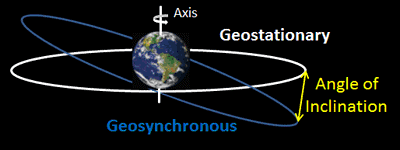##### Key features
• Not in equatorial plane i.e. directly above the equator, it’s in inclined orbit
• Angular velocity of the satellite is equal to angular velocity of earth
• Period of revolution is equal to period of rotation of earth.
• Nearly at an altitude of 36000 Km from earth surface
• There are many geosynchronous orbits used for communication, weather monitoring & surveillance

#### Geostationary orbit / Geosynchronous equatorial orbit

• A circular orbit above the Earth’s equator, following the direction of the Earth’s rotation; has an orbital period equal to the Earth’s rotational period (one sidereal day), and thus appears motionless, at a fixed position in the sky, to ground observers.
• Speed at which a satellite orbits the Earth coincides with the speed that the Earth turns and at the same latitude, however, this does not mean that the satellite and the Earth are traveling at the same speed, but rather that the satellite is traveling fast enough so that its orbit matches the Earth’s rotation.
• When a satellite is in geostationary orbit, its instruments are looking at a certain part of the Earth. That part of the Earth is called a footprint for ex. India
• Suitable for communication satellites, or meteorological (weather) satellites

##### Key features
• Circular orbit + in equatorial plane i.e. directly above the equator and thus inclination is zero
• Geostationary orbit, therefore, is really just a special type of equatorial orbit
• Angular velocity of the satellite is equal to angular velocity of earth
• Period of revolution is equal to period of rotation of earth
• Nearly at an altitude of 36000 Km from earth surface
• A subset of geosynchronous orbit
• There is ONLY one geostationary orbit

### Polar Orbit

• A polar orbit usually has an inclination of 90 degrees to the equator
• On every pass around the Earth, it passes over both the north and south poles
• This allows the satellite to see virtually every part of the Earth as the Earth rotates underneath it
• Suitable for mapping or surveillance operations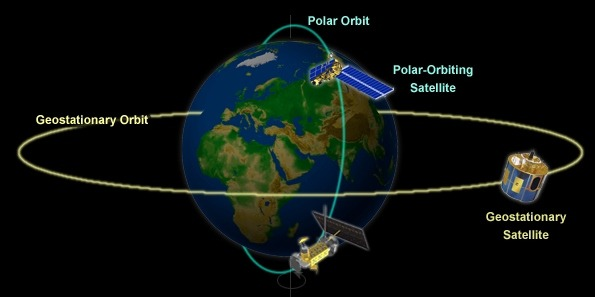#### Sun Synchronous Orbit

• This orbit is a special case of the polar orbit.
• Like a polar orbit, the satellite travels from the north to the south poles
• Since there are 365 days in a year and 360 degrees in a circle, it means that the satellite has to shift its orbit by approximately one degree per day.
• Hence, orbit changes slowly in time with the planet moving around the Sun, and in time with the planet’s rotation so that the spacecraft is always at the same angle to the Sun
• These orbits allow a satellite to pass over a section of the Earth at the same time every day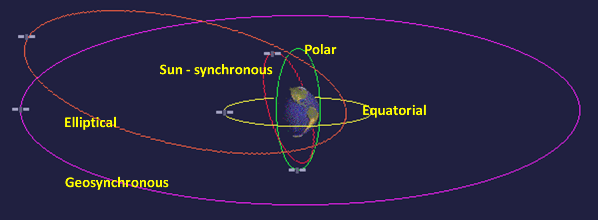#### Elliptical Orbit

• An elliptical orbit, also called an eccentric orbit, is in the shape of an ellipse
• Elliptical orbits are varying in speed & have eccentricity between zero to one
• Satellite’s velocity changes & depends on where it is in its orbital path
• When the satellite is in the part of its orbit closest to the Earth, it moves faster because the Earth’s gravitational pull is stronger.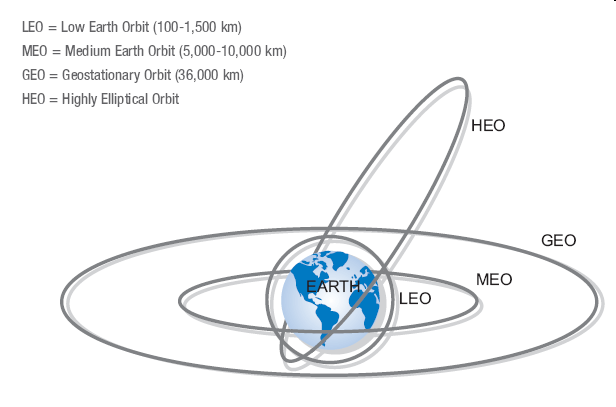• The satellite is moving the fastest at the low point of an elliptical orbit viz. perigee
• The high point of the orbit, when the satellite is moving the slowest, is apogee

## 1 Comment

1.super